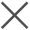• 机器数据分析平台 > 使用文档 > 应用平台 > Pandora机器学习工具包 >fit的使用方法详述 > 数据聚类【聚类】 > 密度聚类算法 - DBSCAN

# 密度聚类算法 - DBSCAN

最近更新时间: 2022-02-24 17:03:07

DBSCAN是一种比较有代表性的基于密度的聚类算法，它假定类别可以通过样本分布的紧密程度决定，同一类别的样本，他们之间肯定是紧密相连的，而不同类别的样本，距离会相对比较远。而DBSCAN就是基于这样的假设，将足够密度的区域划分为一个簇，实现聚类的目的。不支持模型保存

语法：

``````...|fit DBSCAN eps=<float> min_samples=<int> algorithm=<auto (default)| ball_tree | kd_tree | brute> metric=<str> p=<float> <feature_field_1> <feature_field_2>...
``````
• eps参数，用来指定邻域的距离阈值，和样本距离超过eps距离的样本点不在邻域内。如不提供，默认值为0.5。eps越大，越多的点落在核心对象的邻域，则类别数可能会减少。反之，类别数会增多。

• min_samples参数，用来指定即样本点要成为核心对象所需要的𝜖-邻域的样本数阈值。如不提供，默认值为5。在eps一定的情况下，min_samples越大，核心对象越少，类别数越多。反之，类别数会减少。

• algorithm用来指定用来计算最近邻居的算法

• algorithm=ball_tree，使用BallTree
• algorithm=kd_tree，使用KDTree
• algorithm=brute，使用暴力算法
• algorithm=auto（默认值），尝试用实际fit的数值决定最适合的算法
• metric用来指定计算邻居间距离的方法，根据algorithm不同，适用的metric方法也有不同，可以匹配的metric可参考Sci-kit Learn支持的距离衡量方法

• algorithm=ball_tree的情况下，比较常用的metric有：

• cityblock，使用曼哈顿距离（～metric=l1, metric=manhattan)
• euclidean，使用欧式距离（～metric=l2）
• l2，使用欧式距离（～metric=euclidean）
• l1，使用曼哈顿距离（～metric=cityblock, metric=manhattan)
• manhattan，使用曼哈顿距离（～metric=l1, metric=cityblock)
• chebyshev, 使用切比雪夫距离
• cosine，使用余弦相似度
• mahalanobis，使用马氏距离，必须提供>=2个特征字段
• minkowski（默认值），使用闵氏距离，必须同时提供p参数
• algorithm=kd_Tree，比较常用的metric有：

• cityblock，使用曼哈顿距离（～metric=l1, metric=manhattan)
• euclidean，使用欧式距离（～metric=l2）
• l2，使用欧式距离（～metric=euclidean）
• l1，使用曼哈顿距离（～metric=cityblock, metric=manhattan)
• manhattan，使用曼哈顿距离（～metric=l1, metric=cityblock)
• chebyshev, 使用切比雪夫距离
• cosine，使用余弦相似度
• minkowski（默认值），使用闵氏距离，必须同时提供p参数
• algorithm=brute的情况下，比较常用的metric有：

• cityblock，使用曼哈顿距离（～metric=l1, metric=manhattan)
• euclidean，使用欧式距离（～metric=l2）
• l2，使用欧式距离（～metric=euclidean）
• l1，使用曼哈顿距离（～metric=cityblock, metric=manhattan)
• manhattan，使用曼哈顿距离（～metric=l1, metric=cityblock)
• chebyshev, 使用切比雪夫距离
• cosine，使用余弦相似度
• mahalanobis，使用马氏距离，必须提供>=2个特征字段
• minkowski（默认值），使用闵氏距离
• seuclidean，使用平方欧式距离
• p用来指定metric=minkowski的情况下的p参数。若不提供，则默认为2。p必须为正整数。

• p=1，约等于使用曼哈顿距离（l1）
• p=2（默认值），约等于使用欧式距离（l2）
• p=其他正整数，即使用L-p的闵氏距离
以上内容是否对您有帮助？
•